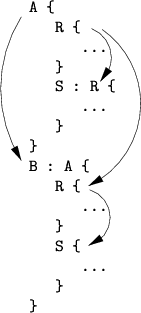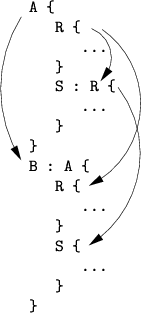### 3.5.1.4 Inheritance Scheme

The inheritance mechanism of the Input Deck database allows for inheritance of every section, subsection, and so on. Since the inheritance scheme is very flexible it had to be well defined otherwise ambiguities would emerge.

The necessity of this is shown for the example below. Section S in Section A inherits from Section R. Modifications in Section R immediately take place in Section S too, because a dynamic approach of data representation is used. Section A with all its entities and their properties are passed on to Section B. Thus, Section B also has two subsections, R and S. Any modification performed in Section R of Section A will even influence the contents of Section B.

```A
{  R
{  // ...
}
S : R
{  // ...
}
}
B : A
{  R
{  // ...
}
S
{  // ...
}
}
```

Figure 3.7: Inheritance schemes.(a)  Implicit inheritance scheme.(b)  Explicit inheritance scheme.

Ambiguity arises between Section S of Section A and Section S of Section B. This is depicted in Fig. 3.7(a) and Fig. 3.7(b) which show the implicit and explicit inheritance schemes, respectively. With implicit inheritance the inheritance information of all subsections is passed on to the successor, whereas with explicit inheritance only the inheritance rules given explicitly by the user are applied. In the first case Section S in Section B is inherited from Section R in Section B - the inheritance scheme is defined in Section A and was passed to Section B. In the second case Section S in Section B does not contain any explicit inheritance information. Since Section B is inherited from Section A, that Section S is inherited from Section S of Section A.

Modifications to the Section S in Section A will therefore influence the Section S of Section B only in the case of explicit inheritance. In the Input Deck database the explicit inheritance scheme has been implemented for the following reason: Be Section A the world, Section R is an ape, and Section S which is derived from Section R is a human. If Section B is derived from Section A to create another world its Section R is again an ape and Section S again a human. This would not be the case with implicit inheritance. Section R of the new world would be an ape again but Section S something else derived from an ape.

Due to the dynamic approach of data representation database queries are not trivial: To find an entry the Input Deck database has to generate a sequence of possible names. To find the variable `~`.B.S.foo in the hierarchy

```A
{  R
{  foo = 1;
}
S : R;
}
B : A;
```

the following sequence is generated:

```~.B.S.foo   // -> does not exist
~.A.S.foo   // -> does not exist
~.A.R.foo   // -> found
```

Finally the existing keyword `~`.A.R.foo is found. During evaluation of expressions such sequences are generated for each variable reference used inside the expressions. Therefore the Input Deck database uses a sophisticated algorithm for creating and representing names which is explained in the following section.

Robert Klima 2003-02-06